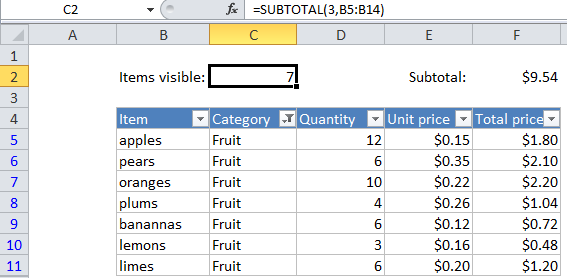## Excel Office

Excel How Tos, Tutorials, Tips & Tricks, Shortcuts

# Count visible rows in a filtered list in Excel

This tutorial shows how to Count visible rows in a filtered list in Excel using the example below;

## Formula

`=SUBTOTAL(3,range)`## Explanation

If you want to count the number of visible items in a filtered list, you can use the SUBTOTAL function, which automatically ignores rows that are hidden by a filter.

Worked Example:   Sum visible rows in a filtered list in Excel

The SUBTOTAL function can perform calculations like COUNT, SUM, MAX, MIN, and more. (For a full list, see the table here). What makes SUBTOTAL especially interesting and useful is that it automatically ignores items that are not visible in a filtered list or table. This makes it ideal for showing how many items are visible in a list, the subtotal of visible rows, etc.

Worked Example:   Count visible rows only with criteria in Excel

Following the example in the image above, to count the number of non-blank rows visible when a filter is active, use:

`=SUBTOTAL(3,B5:B14)`

If you are hiding rows manually (i.e. right-click, Hide), and not using the auto-filter, use this version instead:

`=SUBTOTAL(103,B5:B14))`
Worked Example:   Sum visible rows in a filtered list in Excel